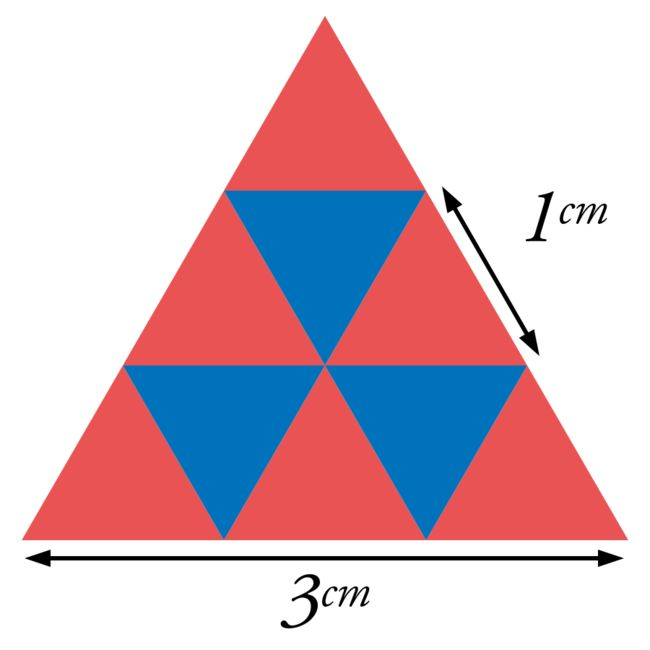# Triangle Trouble

Geometry Level 3The diagram shows an equilateral triangle of side length $3\text{ cm}$, made by tessellating smaller equilateral triangles of side length $1\text{ cm}$. How many such $1\text{ cm}$ triangles would a larger equilateral triangle of side length $1.25\text{ m}$ contain?

×